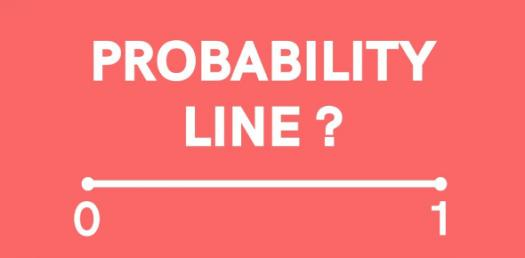# Probability Practice Test Questions! Quiz

10 Questions | Attempts: 640SettingsProbability is a basic idea for statistics, metrology risk analysis, and game theory, and it comes up quite often in other fields. Probability is the study of the unpredictable sequence of events. The theoretical probability is the number of outcomes of the event divided by the number of possible outcomes. Taking this quiz will tell have what it takes to practice probability.

• 1.
A bag contains 8 red beads, 14 green beads and x blue beads. If a bead is picked at random from the bag, the probability of picking a green bead is 1/3. Find the value of x.
• A.

20

• B.

22

• C.

23

• D.

25

• 2.
A box contains with 27 Mathematics and Science books. If a book is selected at random from the box, the probability of selecting a Science book is 4/9. Then 3 Mathematics books and 2 Science books are added into the box. A book is selected at random from the box. Find the probability of selecting a Mathematics book.
• A.

15/32

• B.

17/32

• C.

5/9

• D.

9/16

• 3.
A container contains sweets of orange, coffee and pineapple flavours. A child picks one sweet at random from the container. The probabilities of him choosing a sweet with an orange flavour and a sweet with a coffee flavour are 2/9 and 1/3 respectively. If the number of sweets with pineapple flavour is 160, find the total number of sweets in the container.
• A.

450

• B.

360

• C.

315

• D.

270

• 4.
A box contains 24 green T-shirts and some red T-shirts. A T-shirt is chosen at random from the box. The probability of choosing a green T-shirt is 3/8.  Find the number of red T-shirts in the box.
• A.

25

• B.

30

• C.

35

• D.

40

• 5.
A number is chosen at random from set S = {3, 5, 9, 12, 16, 25, 27, 29}. Find the probability that the number chosen is a perfect square.
• A.

3/8

• B.

1/2

• C.

3/4

• D.

5/8

• 6.
In a group, 350 of the 450 workers are female. Then 50 male workers join the group. A worker is chosen at random from the group. Find the probability of choosing a male worker.
• A.

2/9

• B.

7/9

• C.

3/10

• D.

1/10

• 7.
There are 56 red ink pens and a number of black ink pens in a box. The probability that a black ink pen is chosen at random from the box is 3/7. How many black ink pens in the box?
• A.

42

• B.

49

• C.

60

• D.

98

• 8.
There are 24 Mathematics books, 30 Science books and 36 History books in a book rack. A book is picked at random from the rack.  The probability of picking a Mathematics book is
• A.

1/4

• B.

4/15

• C.

1/3

• D.

2/5

• 9.
Faizah receives 36 Hari Raya greeting cards from overseas and 56 greeting cards sent locally. One third of the overseas cards and three quarters of the local cards are from her relatives. If Faizah picks a greeting card at random, what is the probability that the greeting card is not from her relatives?
• A.

27/46

• B.

11/12

• C.

19/46

• D.

12/13

• 10.
A basket contains 15 bottles of flavoured-orange drink and vanilla-flavoured drink. If a bottle is selected at random from the basket, the probability of selecting a bottle of orange-flavour drink is 3/5. Then five bottles of orange-flavoured drink are added into the basket. If a bottle is selected at random from the basket, find the probability of selecting a bottle of vanilla-flavour drink.
• A.

1/5

• B.

2/5

• C.

3/10

• D.

7/10

## Related TopicsBack to top
×

Wait!
Here's an interesting quiz for you.